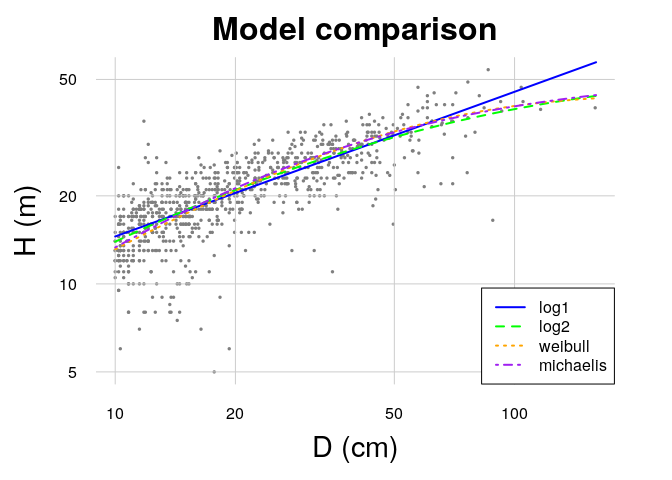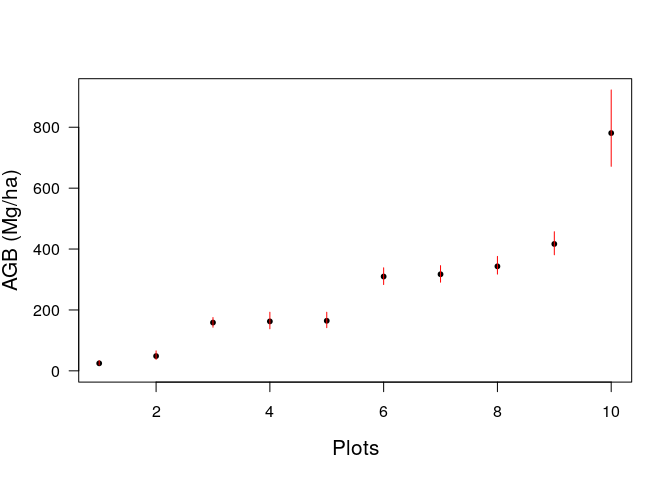# 1 Load BIOMASS and datasets

Install BIOMASS (to be done once)

install.packages("BIOMASS")

require(BIOMASS)
require(knitr) # To build tables in this document

Load the two datasets stored in the package

data(KarnatakaForest)
str(KarnatakaForest)
## 'data.frame':    65889 obs. of  8 variables:
##  $plotId : chr "BSP1" "BSP1" "BSP1" "BSP1" ... ##$ treeId : chr  "BSP1_1" "BSP1_2" "BSP1_3" "BSP1_4" ...
##  $family : chr "Fabaceae" "Fabaceae" "Fabaceae" "Fabaceae" ... ##$ genus  : chr  "Acacia" "Acacia" "Acacia" "Acacia" ...
##  $species: chr "sinuata" "sinuata" "sinuata" "sinuata" ... ##$ D      : num  3.82 4.14 4.14 4.46 4.46 ...
##  $lat : num 14.4 14.4 14.4 14.4 14.4 ... ##$ long   : num  74.9 74.9 74.9 74.9 74.9 ...
#
data(NouraguesHD)
str(NouraguesHD)
## 'data.frame':    1051 obs. of  7 variables:
##  $plotId : chr "Plot1" "Plot1" "Plot1" "Plot1" ... ##$ genus  : chr  "indet" "Qualea" "Dicorynia" "Protium" ...
##  $species: chr "indet" "rosea" "guianensis" "cf_guianense" ... ##$ D      : num  11.5 11.6 83.9 15 36.8 13.5 17.8 17.8 15.9 17.8 ...
##  $H : num 12 16 40 18 27 20 24 21 22 24 ... ##$ lat    : num  4.07 4.07 4.07 4.07 4.07 ...
##  $long : num -52.7 -52.7 -52.7 -52.7 -52.7 ... Select 10 plots for illustrative purpose selecPlot <- KarnatakaForest$plotId %in% c("BSP2", "BSP12", "BSP14", "BSP26", "BSP28", "BSP30", "BSP34", "BSP44", "BSP63", "BSP65")
KarnatakaForestsub <- droplevels(KarnatakaForest[selecPlot, ])

# 2 Retrieve wood density

## 2.1 Check and retrieve taxonomy

First, check for any typo in the taxonomy

Taxo <- correctTaxo(genus = KarnatakaForestsub$genus, species = KarnatakaForestsub$species, useCache = T, verbose = F)
KarnatakaForestsub$genusCorr <- Taxo$genusCorrected
KarnatakaForestsub$speciesCorr <- Taxo$speciesCorrected

If needed, retrieve APG III families and orders from genus names

APG <- getTaxonomy(KarnatakaForestsub$genusCorr, findOrder = T) KarnatakaForestsub$familyAPG <- APG$family KarnatakaForestsub$orderAPG <- APG$order ## 2.2 Wood density Retrieve wood density using the plot level average if no genus level information is available dataWD <- getWoodDensity( genus = KarnatakaForestsub$genusCorr,
species = KarnatakaForestsub$speciesCorr, stand = KarnatakaForestsub$plotId
)
## The reference dataset contains 16467 wood density values
## Your taxonomic table contains 196 taxa

The same but using the family average and adding other wood density values as references (here invented for the example)

LocalWoodDensity <- data.frame(
genus = c("Ziziphus", "Terminalia", "Garcinia"),
species = c("oenopolia", "bellirica", "indica"),
wd = c(0.65, 0.72, 0.65)
)

dataWD <- getWoodDensity(
genus = KarnatakaForestsub$genusCorr, species = KarnatakaForestsub$speciesCorr,
family = KarnatakaForestsub$familyAPG, stand = KarnatakaForestsub$plotID,
)
## The reference dataset contains 16470 wood density values
## Your taxonomic table contains 196 taxa

Below the number of wood density value estimated at the species, genus and plot level:

# At species level
sum(dataWD$levelWD == "species") ##  2412 # At genus level sum(dataWD$levelWD == "genus")
##  2960
# At plot level
sum(!dataWD$levelWD %in% c("genus", "species")) ##  394 # 3 Build height-diameter models You may compare different models at once result <- modelHD( D = NouraguesHD$D,
H = NouraguesHD$H, useWeight = TRUE ) ## To build a HD model you must use the parameter 'method' in this functionkable(result) method color RSE RSElog Average_bias log1 blue 4.305059 0.2231136 0.0042275 log2 green 4.222718 0.2215495 0.0031217 weibull orange 4.307951 NA 0.0028240 michaelis purple 4.294488 NA 0.0145642 Compute the local H-D model with the lowest RSE HDmodel <- modelHD( D = NouraguesHD$D,
H = NouraguesHD$H, method = "log2", useWeight = TRUE ) Compute models specific to given stands HDmodelPerPlot <- modelHD( D = NouraguesHD$D, H = NouraguesHD$H, method = "weibull", useWeight = T, plot = NouraguesHD$plotId
)
ResHD <- t(sapply(HDmodelPerPlot, function(x) c(coef(x$model), RSE = x$RSE)))
kable(ResHD, row.names = T, digits = 3)
a b c RSE
Plot1 43.673 29.629 0.987 4.503
Plot2 369.917 46732.555 0.367 3.865

# 4 Retrieve height data

Retrieve height data from a local Height-diameter model (Note that using a HD model built on French guianan trees for Indian trees is only for illustrative purpose here)

dataHlocal <- retrieveH(
D = KarnatakaForestsub$D, model = HDmodel ) Retrieve height data from a Feldpaush et al. (2012) averaged model dataHfeld <- retrieveH( D = KarnatakaForestsub$D,
region = "SEAsia"
)

Retrieve height data from Chave et al. (2012) equation 6

dataHchave <- retrieveH(
D = KarnatakaForestsub$D, coord = KarnatakaForestsub[, c("long", "lat")] ) # 5 Estimate AGB Organize data KarnatakaForestsub$WD <- dataWD$meanWD KarnatakaForestsub$H <- dataHlocal$H KarnatakaForestsub$Hfeld <- dataHfeld$H Compute AGB(Mg) per tree AGBtree <- computeAGB( D = KarnatakaForestsub$D,
WD = KarnatakaForestsub$WD, H = KarnatakaForestsub$H
)

Compute AGB(Mg) per plot

AGBplot <- summaryByPlot(AGBtree, KarnatakaForestsub$plotId) Compute AGB(Mg) per tree without height information (Eq. 7 from Chave et al. (2014)) AGBplotChave <- summaryByPlot( computeAGB( D = KarnatakaForestsub$D, WD = KarnatakaForestsub$WD, coord = KarnatakaForestsub[, c("long", "lat")] ), KarnatakaForestsub$plotId
)

Compute AGB(Mg) per tree with Feldpausch et al. (2012) regional H-D model

AGBplotFeld <- summaryByPlot(
computeAGB(
D = KarnatakaForestsub$D, WD = KarnatakaForestsub$WD,
H = KarnatakaForestsub$Hfeld ), plot = KarnatakaForestsub$plotId
)

# 6 Propagate AGB errors

Organize data

KarnatakaForestsub$sdWD <- dataWD$sdWD
KarnatakaForestsub$HfeldRSE <- dataHfeld$RSE

Propagate error for all tree at once using the local HD model constructed above (modelHD), i.e. non-independent allometric errors will be assigned to all trees at each iteration, independently of plots.

resultMC <- AGBmonteCarlo(D = KarnatakaForestsub$D, WD = KarnatakaForestsub$WD, errWD = KarnatakaForestsub$sdWD, HDmodel = HDmodel, Dpropag = "chave2004") Res <- summaryByPlot(resultMC$AGB_simu, KarnatakaForestsub$plotId) Res <- Res[order(Res$AGB), ]
plot(Res$AGB, pch = 20, xlab = "Plots", ylab = "AGB (Mg/ha)", ylim = c(0, max(Res$Cred_97.5)), las = 1, cex.lab = 1.3)
segments(seq(nrow(Res)), Res$Cred_2.5, seq(nrow(Res)), Res$Cred_97.5, col = "red")Using the Feldpaush regional HD averaged model (code only given)

resultMC <- AGBmonteCarlo(
D = KarnatakaForestsub$D, WD = KarnatakaForestsub$WD,
errWD = KarnatakaForestsub$sdWD, H = KarnatakaForestsub$Hfeld,
errH = KarnatakaForestsub$HfeldRSE, Dpropag = "chave2004" ) Res <- summaryByPlot(resultMC$AGB_simu, KarnatakaForestsub$plotId) Res <- Res[order(Res$AGB), ]
plot(Res$AGB, pch = 20, xlab = "Plots", ylab = "AGB (Mg/ha)", ylim = c(0, max(Res$Cred_97.5)), las = 1, cex.lab = 1.3)
segments(seq(nrow(Res)), Res$Cred_2.5, seq(nrow(Res)), Res$Cred_97.5, col = "red")

Per plot using the Chave et al. (2014) Equation 7 (code only given)

resultMC <- AGBmonteCarlo(
D = KarnatakaForestsub$D, WD = KarnatakaForestsub$WD,
errWD = KarnatakaForestsub$sdWD, coord = KarnatakaForestsub[, c("long", "lat")], Dpropag = "chave2004" ) Res <- summaryByPlot(resultMC$AGB_simu, KarnatakaForestsub$plotId) Res <- Res[order(Res$AGB), ]
plot(Res$AGB, pch = 20, xlab = "Plots", ylab = "AGB (Mg/ha)", ylim = c(0, max(Res$Cred_97.5)), las = 1, cex.lab = 1.3)
segments(seq(nrow(Res)), Res$Cred_2.5, seq(nrow(Res)), Res$Cred_97.5, col = "red")

# 7 Some tricks

## 7.1 Mixing measured and estimated height values

If you want to use a mix of directly-measured height and of estimated ones, you may do the following steps.

1. Build a vector of H and RSE where we assume an error of 0.5 m on directly measured trees
NouraguesHD$Hmix <- NouraguesHD$H
NouraguesHD$RSEmix <- 0.5 filt <- is.na(NouraguesHD$Hmix)
NouraguesHD$Hmix[filt] <- retrieveH(NouraguesHD$D, model = HDmodel)$H[filt] NouraguesHD$RSEmix[filt] <- HDmodel$RSE 1. Apply the AGBmonteCarlo by setting the height values and their errors (which depend on wether the tree was directly measured or estimated) wd <- getWoodDensity(NouraguesHD$genus, NouraguesHD$species) resultMC <- AGBmonteCarlo( D = NouraguesHD$D, WD = wd$meanWD, errWD = wd$sdWD,
H = NouraguesHD$Hmix, errH = NouraguesHD$RSEmix,
Dpropag = "chave2004"
)
Res <- summaryByPlot(resultMC$AGB_simu, NouraguesHD$plotId)
Res <- Res[order(Res$AGB), ] plot(Res$AGB, pch = 20, xlab = "Plots", ylab = "AGB (Mg/ha)", ylim = c(0, max(Res$Cred_97.5)), las = 1, cex.lab = 1.3) segments(1:nrow(Res), Res$Cred_2.5, 1:nrow(Res), Res\$Cred_97.5, col = "red")